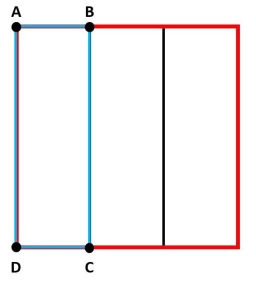# Rectangular 81233

A rectangular strip of paper measuring 4 cm x 13 cm is folded as shown. The two resulting rectangles have areas P and Q, where P = 2Q. Calculate the value of x.

Note divide the side of 13 cm by x and 13-x.

x =  4.3333 cm

### Step-by-step explanation:Did you find an error or inaccuracy? Feel free to write us. Thank you!

Tips for related online calculators
Check out our ratio calculator.

#### Grade of the word problem:

We encourage you to watch this tutorial video on this math problem: